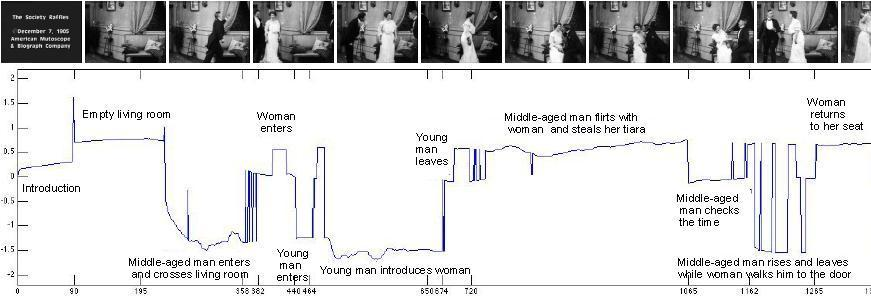Identification of Hybrid Systems
Project Summary
Over the past decade, we have witnessed tremendous advances on the analysis, verification, stability and controllability of hybrid systems, which are dynamical systems that exhibit interacting continuous and discrete behavior. However, these advances are strongly based on the assumption that accurate quantitative models are readily available. Relatively less attention has been paid to the more difficult inverse problem: given input-output data generated by a hybrid system, identify the number of discrete states, the hybrid state (continuous and discrete) and the model parameters associated with each state. Such identification problems arise in a variety of disciplines, including control, robotics, bioengineering, computer vision and machine learning, in which accurate models of complex physical processes are often difficult to obtain from first principles and only measured data is obtainable. In our research, we focus on both batch and recursive identification of switched autoregressive exogenous (SARX) systems.

The key to our approach is to view the identification of multiple ARX models as the identification of a single, though more complex, lifted dynamical model built by applying a polynomial embedding to the input/output data. We show that the dynamics of this lifted model do not depend on the value of the discrete state or the switching mechanism, and are linear on the so-called hybrid model parameters. Therefore, one can identify the parameters of the lifted model using a standard ARX identifier applied to the embedded input/output data. The estimated hybrid model parameters are then used to build a polynomial whose derivatives at a regressor give an estimate of the parameters of the ARX model generating that regressor. Specific details for the batch and recursive methods are given below.

Batch Identification of Switched ARX Models
We propose an algebraic geometric solution to the identification of linear SARX systems. We show that the identification of the model parameters can be decoupled from the inference of the hybrid state and the switching mechanism generating the transitions, hence we do not constraint the switches to be separated by a minimum dwell time. The decoupling is obtained from the so-called hybrid decoupling constraint, which establishes a connection between linear hybrid system identification, polynomial factorization and hyper-plane clustering. In essence, we represent the number of discrete states n as the degree of a homogeneous polynomial p and the model parameters as factors of p. We then show that one can estimate n from a rank constraint on the data, the coefficients of p from a linear system, and the model parameters from the derivatives of p.
Recursive Identification of Switched ARX Models
In many real time applications, the data are not entirely available and hence, need to be processed recursively. Therefore, in , , and  we developed a recursive method for the identification of SARX models, under the assumption that the number of models, the model orders and the mode sequence are unknown. The key to our approach is to view the identification of multiple ARX models as the identification of a single, though more complex, lifted dynamical model built by applying a polynomial embedding to the input/output data. We show that the dynamics of this lifted model do not depend on the value of the discrete state or the switching mechanism, and are linear on the so-called hybrid model parameters. Therefore, one can identify the parameters of the lifted model using a standard recursive identifier applied to the embedded input/output data. The estimated hybrid model parameters are then used to build a polynomial whose derivatives at a regressor give an estimate of the parameters of the ARX model generating that regressor. The estimated ARX model parameters are shown to converge exponentially to their true values under a suitable persistence of excitation condition on a projection of the embedded input/output data.
Application to Video Segmentation
In order to test the performance of our approach on real data, we apply our algorithm to temporal video segmentation in computer vision. This problem consists of separating a video sequence into multiple scenes, where each scene consisting of a group of frames with similar content. For this purpose, we model the dynamics of the video sequence within a scene with an ARX model. Thus the entire video can be described as the output of a SARX model, where each mode correspond to a different scene in the video.Publications

Automatica, 2007 (Accepted).

IEEE Conference on Decision & Control, pages 6115-6121, 2005.

Hybrid Systems: Computation and Control, pages 449-465. 2005.

IEEE Conference on Decision & Control, pages 32-37, 2004.

IEEE Conference on Decision and Control, pages 167-172, December 2003.